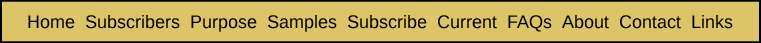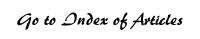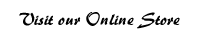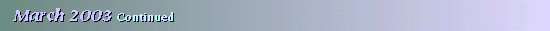Technical Addendum— An ELS Extension Model This technical addendum describes the derivation of the formulae used to determine the expected number of extended ELSs (with varying numbers of extensions) to emerge from a search process beginning with n initial ELSs. We shall denote initial ELSs by the symbol “I.” After a Hebrew expert examines the string of letters resulting from taking every j-th letter before and after the initial ELS with a skip of j letters, one of two results will occur in each instance that there is an opportunity to find an extension of the initial ELS. If no extension is found, this will be denoted by “N” and if one is found, it will be denoted by “E.” The following chart presents the complete range of outcomes from this process up through the location of four extensions to an initial ELS. From this chart, the formula for determining the expected number of ELSs with a given number of extensions will become evident, preventing the need to extend the chart out to the right to incorporate additional outcomes where five or more extensions have been found. Each outcome is represented first by a combination of the letters I, E and N, indicating the order in which things appeared in that outcome. Immediately below that outcome is a formula for the expected number of ELSs that will conform to that description. In that formula, n is the total number of initial ELSs, a is the probability of finding a grammatically correct Hebrew extension of the preceding ELS, and b is the probability of not finding an extension. Obviously, b is the complement of a, so b = 1 – a. However, we used b in the table below to shorten the formulae, and make the derivation of each formula easier to understand.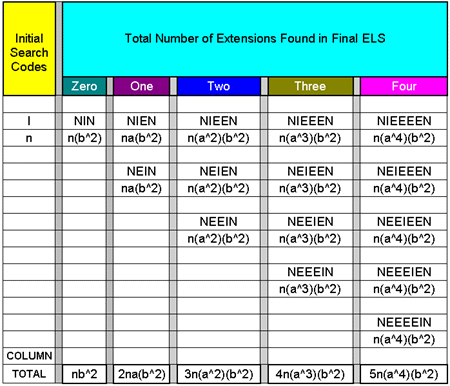The beginning of the search process is represented by the leftmost column of the table, where there are n instances where I, an initial ELS, appears. Each successive step in the search process is represented by a new column immediately to the right of the earlier columns. The only way that zero extensions will be found is the situation where no extension is found either before or after the initial ELS. This is denoted by “NIN.” The probability of this occurring for any given initial ELS is b^2. So the expected number of final ELSs that have no extension will be n(b^2). There are two ways that a final ELS can have one and only one extension. The first occurs when the extension appears after the initial ELS (NIEN) and the second when the extension appears before the initial ELS (NEIN). It should be noted that we are using English conventions here, proceeding to read from left to right, for the sake of most of our readers—rather than the Hebrew convention, where reading is done from right to left. The total expected number of final ELSs to emerge from the first way of having exactly one extension is na(b^2), as is the case for the second way. So the total expected number of final ELSs with exactly one extension will be 2na(b^2), as shown at the bottom of the “One” column. There are three ways that a final ELS can have exactly two extensions. The first occurs when both extensions appear after the initial ELS (NIEEN). The second occurs when an extension appears on both sides of the initial ELS (NEIEN). The third occurs when both extensions appear before the initial ELS (NEEIN). The total expected number of final ELSs to emerge from the first way of having exactly one extension is n(a^2)(b^2), as is the case for the second and the third way. So the total expected number of final ELSs with exactly two extensions will be 3n(a^2)(b^2), as shown at the bottom of the “Two” column. From the patterns evident in the above table we can see that the total expected number of final ELSs with exactly k extensions will be (k+1)n(a^k)(b^2). Since n and b^2 appear in each term, we can factor them out of an expression for the total number of final ELSs, to get (1) n(b^2) [Σ(k+1)(a^k)], where k ranges from 0 to infinity. If we multiply each term of this power series by a in both the numerator and denominator, we obtain {[n(b^2)]/a} [Σ(k+1)(a^{k+1})]. If we shift the value of (k+1) by one for each term, so that the series is summed from k = 1 to infinity, rather than from k = 0 to infinity, it becomes {[n(b^2)]/a} [Σ k(a^k)]. According to formula 40 on page 8 of Summation of Series, collected by L.B.W. Jolley: [Σ n(x^n) = x/[(1-x)^2], where x < 1, and the series is summed from n=1 to infinity. If we substitute k for n and a for x in this formula, we have [Σ k(a^k) = a/[(1-a)^2] . By substituting the simple expression on the right for the power series expression in (1) above, we get {[n(b^2)]/a} [a/[(1-a)^2]] . Since b = (1-a), this expression becomes {[n(1-a)^2)]/a} [a/[(1-a)^2]] = n. So this total expression equals n, as it must, since n final ELSs will emerge from searching n initial ELSs. Why is it that the total expected number of final ELSs with exactly k extensions is given by (k+1)n(a^k)(b^2)? This formula may be broken down into two parts, the first being n, the total number of opportunities to examine extensions around an initial ELS, times the rest of the expression, which is the probability of getting exactly k extensions, given the opportunity of examining one letter string centered around one initial ELS. [Total Expected Extensions] = [Total Opportunities] x [Probability of the Specified Number of Extensions Occurring, Given One Opportunity] In order to get exactly k extensions, each possible way of doing that involves k extensions and 2 instances of not finding any extension. The probability of the former is a^k and of the latter, b^2. As it turns out, there are exactly (k+1) ways of getting k extensions. To see this let us look at a few specific examples. There are three ways to get two extensions, and they are denoted by NIEEN, NEIEN & NEEIN. The first way occurs when the I appears prior to both E’s. The second occurs when the I appears in between the two E’s, and the third occurs when the I appears after the two E’s. In all cases, there is an N at both ends. There are three possible locations for the I in between the two N’s. It is either in the first, second or third position. There are three positions because there must be two E’s and one I. Hence, for k extensions, there are (k+1) ways of arriving at that result. Return to Head to Head Comparison article

Codes Seminar Set for April 29

The Horizon Institute will be presenting a free seminar on Bible codes Tuesday, April 29, 7 p.m., at the Red Lion Hotel, Medford, Oregon.

Bible Codes: Fact or Fiction will feature speakers Ed Sherman, president of the Isaac Newton Bible Code Research Society, Dr. Nathan Jacobi, our Hebrew expert, and Dave Swaney, editor of Bible Code Digest.
 | HOME | SUBSCRIBERS ONLY | OUR PURPOSE | FREE SAMPLES | CURRENT | | FAQs | ABOUT BIBLE CODES | SUBSCRIBE | CONTACT | Copyright © 2016 BibleCodeDigest.com